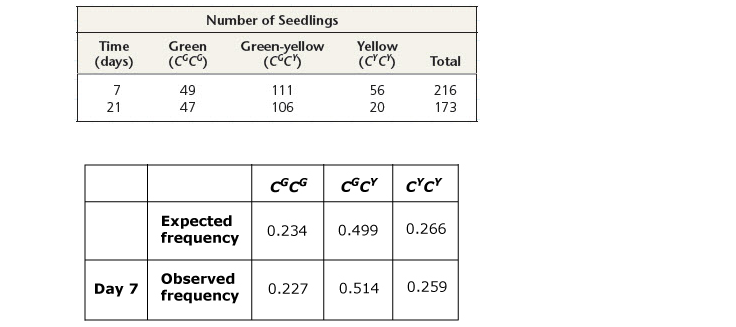# Problem: Compare the observed genotype frequencies you just calculated with the genotype frequencies expected if the population is in Hardy-Weinberg equilibrium. At day 7, is the seedling population in Hardy-Weinberg equilibrium, or is evolution occurring?

###### Problem Details

Compare the observed genotype frequencies you just calculated with the genotype frequencies expected if the population is in Hardy-Weinberg equilibrium. At day 7, is the seedling population in Hardy-Weinberg equilibrium, or is evolution occurring?Frequently Asked Questions

What scientific concept do you need to know in order to solve this problem?

Our tutors have indicated that to solve this problem you will need to apply the Hardy-Weinberg Model concept. You can view video lessons to learn Hardy-Weinberg Model. Or if you need more Hardy-Weinberg Model practice, you can also practice Hardy-Weinberg Model practice problems.

What is the difficulty of this problem?

Our tutors rated the difficulty ofCompare the observed genotype frequencies you just calculate...as high difficulty.

How long does this problem take to solve?

Our expert Biology tutor, Kaitlyn took 7 minutes and 14 seconds to solve this problem. You can follow their steps in the video explanation above.

What professor is this problem relevant for?

Based on our data, we think this problem is relevant for Professor Muehlbauer's class at QC CUNY .